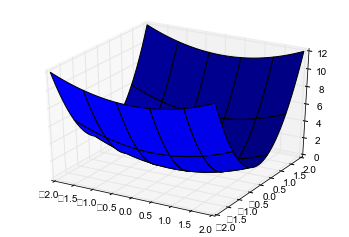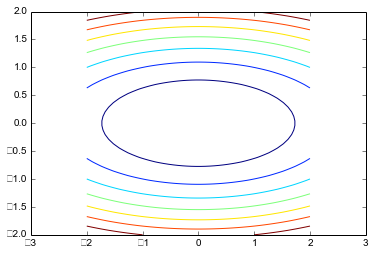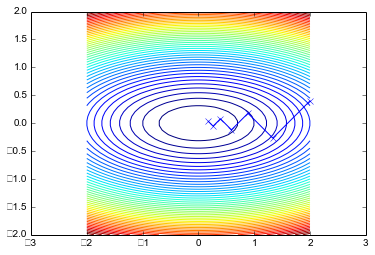# Steepest Descent

In :
import numpy as np
import numpy.linalg as la

import scipy.optimize as sopt

import matplotlib.pyplot as pt
from mpl_toolkits.mplot3d import axes3d


Here's a function. It's an oblong bowl made of two quadratic functions.

This is pretty much the easiest 2D optimization job out there.

In :
def f(x):
return 0.5*x**2 + 2.5*x**2

def df(x):
return np.array([x, 5*x])


Let's take a look at the function. First in 3D:

In :
fig = pt.figure()
ax = fig.gca(projection="3d")

xmesh, ymesh = np.mgrid[-2:2:50j,-2:2:50j]
fmesh = f(np.array([xmesh, ymesh]))
ax.plot_surface(xmesh, ymesh, fmesh)

Out:
<mpl_toolkits.mplot3d.art3d.Poly3DCollection at 0x7f7431a9d978>

/usr/lib/python3/dist-packages/matplotlib/backends/backend_agg.py:517: DeprecationWarning: npy_PyFile_Dup is deprecated, use npy_PyFile_Dup2
filename_or_obj, self.figure.dpi)And then as a "contour plot":

In :
pt.axis("equal")
pt.contour(xmesh, ymesh, fmesh)

Out:
<matplotlib.contour.QuadContourSet at 0x7f74273f6f60>Next, initialize steepest descent with a starting guess:

In :
guesses = [np.array([2, 2./5])]


Next, run Steepest Descent:

In :
x = guesses[-1]
s = -df(x)

def f1d(alpha):
return f(x + alpha*s)

alpha_opt = sopt.golden(f1d)
next_guess = x + alpha_opt * s
guesses.append(next_guess)

print(next_guess)

[ 0.17558299  0.0351166 ]



Here's some plotting code to illustrate what just happened:

In :
pt.axis("equal")
pt.contour(xmesh, ymesh, fmesh, 50)
it_array = np.array(guesses)
pt.plot(it_array.T, it_array.T, "x-")

Out:
[<matplotlib.lines.Line2D at 0x7f74257e43c8>]In []: